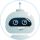# Stars And Galaxies Research Paper Stars

• Subject
• Category
• Topic
• Pages 3
• Words 728
• Views 639
• Pages 3
• Words 728
• Views 639

Get original paper in 3 hours and nail the task

124 experts online

Stars And Galaxies Essay, Research Paper

jects. Part of Newton’s first law, the Law of Inertia, states that objects in motion travel in a straight line at a constant velocity unless they are acted upon by a net force. According to this law, the planets in space should travel in straight lines. However, as early as the time of Aristotle, the planets were known to travel on curved paths. Newton reasoned that the circular motions of the planets are the result of a net force acting upon each of them. That force, he concluded, is the same force that causes an apple to fall to the ground–gravity. Newton’s experimental research into the force of gravity resulted in his elegant mathematical statement that is known today as the Law of Universal Gravitation. According to Newton, every mass in the universe attracts every other mass. The attractive force between any two objects is directly proportional to the product of the two masses being measured and inversely proportional to the square of the distance separating them. If we let F represent this force, r the distance between the centers of the masses, and m1 and m2 the magnitude of the two masses, the relationship stated can be written symbolically as: is defined mathematically to mean “is proportional to.”) From this relationship, we can see that the greater the masses of the attracting objects, the greater the force of attraction between them. We can also see that the farther apart the objects are from each other, the less the attraction. It is important to note the inverse square relationship with respect to distance. In other words, if the distance between the objects is doubled, the attraction between them is diminished by a factor of four, and if the distance is tripled, the attraction is only one-ninth as much. Newton’s Law of Universal Gravitation was later quantified by eighteenth-century English physicist Henry Cavendish who actually measured the gravitational force between two one-kilogram masses separated by a distance of one meter. This attraction was an extremely weak force, but its determination permitted the proportional relationship of Newton’s law to be converted into an equation. This measurement yielded the universal gravitational constant or G.

This essay was written by a fellow student. You may use it as a guide or sample for writing your own paper, but remember to cite it correctly. Don’t submit it as your own as it will be considered plagiarism.

## Need a custom essay sample written specially to meet your requirements?

Choose skilled expert on your subject and get original paper with free plagiarism report

Without paying upfront